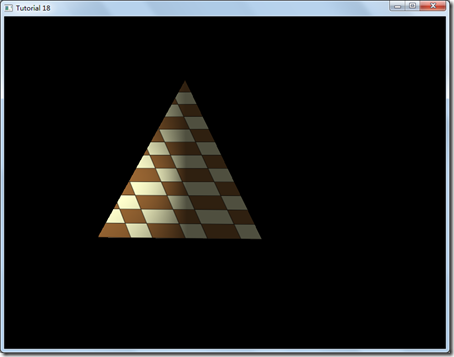#opengl 教程(18) 漫反射光

0
0
01. 云栖社区>
2. 博客>
3. 正文

## opengl 教程(18) 漫反射光

环境光和漫反射光最大的区别在于：漫反射光依赖于光源的方向，而环境光和光源方向完全无关，环境光在场景中是均匀分布的，对场景中的所有物体都有效，而漫反射光在物体朝向光源的一面才有光照效果，在背面则没有光照效果。

如下图所示，除了光源方向，漫反射光还和物体表面的法向有关。

假设上图中2个光束的强度都是一样的，唯一不同的只是方向，则在左边的图中，物体表面光照要亮一些，因为光线是直射，而右边的图中，物体表面光照要弱一些，因为有一个角度。

漫反射光照模型实际上基于Lambert's cosine law，也就是说物体表面反射的光照强度和与光源方向和物体表面法向有关，光源方向和法向的夹角的越小，则物体表面反射的光强越大。 用公式表示就是光源的强度乘以光源和物体表面法向夹角的余弦值。

在上图中，有四道光A,B,C,D,注意：物体表面法向和A重合，但方向相反。光线A和法向的夹角是0，余弦值就是1，所以A光速光照的强度最大，B次之，C和法向夹角是90度，所以光照强度是0，而D作用与物体的背面，余弦值是个负值，光照强度不可能是个负值，所以D的光照强度也是0，也就是说，光源要在物体表面有光照效果，光源方向和物体表面法向夹角必须是0-90度之间。

如果物体表面是个平面，那么法向就是一个固定的向量，但真实物体表面往往并不是平面，所以物体表面的法向是不断变化的，如下图中的两个法向。

#### 主要代码：

lighting_technique.h

`struct DirectionalLight {     Vector3f Color;     float AmbientIntensity;     Vector3f Direction;     float DiffuseIntensity; };`

`layout (location = 0) in vec3 Position; layout (location = 1) in vec2 TexCoord; layout (location = 2) in vec3 Normal; uniform mat4 gWVP; uniform mat4 gWorld; out vec2 TexCoord0; out vec3 Normal0; void main() {     gl_Position = gWVP * vec4(Position, 1.0);     TexCoord0 = TexCoord;     Normal0 = (gWorld * vec4(Normal, 0.0)).xyz; }`

`in vec2 TexCoord0; in vec3 Normal0; out vec4 FragColor; struct DirectionalLight {     vec3 Color;     float AmbientIntensity;     float DiffuseIntensity;     vec3 Direction; };`

`void main() {     vec4 AmbientColor = vec4(gDirectionalLight.Color, 1.0f) *             gDirectionalLight.AmbientIntensity; `

float DiffuseFactor = dot(normalize(Normal0), -gDirectionalLight.Direction);

首先我们会计算环境光的强度，接着计算漫反射光的强度。我们使用光源方向向量的负值点乘像素的法向向量，得到光源和法向夹角的余弦值，最后用光源强度乘以余弦值得到最终的漫反射光强，如果余弦值是负的，那就说明光照在物体的背面，此时漫反射光强为0。

`    vec4 DiffuseColor;     if (DiffuseFactor > 0) {         DiffuseColor = vec4(gDirectionalLight.Color, 1.0f) * gDirectionalLight.DiffuseIntensity * DiffuseFactor;     }     else {         DiffuseColor = vec4(0, 0, 0, 0);     } `

`    FragColor = texture2D(gSampler, TexCoord0.xy) * (AmbientColor + DiffuseColor); }`

lighting_technique.cpp

`void LightingTechnique::SetDirectionalLight(const DirectionalLight& Light) {     glUniform3f(m_dirLightLocation.Color, Light.Color.x, Light.Color.y, Light.Color.z);     glUniform1f(m_dirLightLocation.AmbientIntensity, Light.AmbientIntensity);     Vector3f Direction = Light.Direction;     Direction.Normalize();     glUniform3f(m_dirLightLocation.Direction, Direction.x, Direction.y, Direction.z);     glUniform1f(m_dirLightLocation.DiffuseIntensity, Light.DiffuseIntensity); }`

tutorial18.cpp

`struct Vertex {     Vector3f m_pos;     Vector2f m_tex;     Vector3f m_normal;     Vertex() {}     Vertex(Vector3f pos, Vector2f tex)     {         m_pos = pos;         m_tex = tex;         m_normal = Vector3f(0.0f, 0.0f, 0.0f);     } };`

`void CalcNormals(const unsigned int* pIndices, unsigned int IndexCount, Vertex* pVertices, unsigned int VertexCount) {     for (unsigned int i = 0 ; i < IndexCount ; i += 3) {         unsigned int Index0 = pIndices[i];         unsigned int Index1 = pIndices[i + 1];         unsigned int Index2 = pIndices[i + 2];         Vector3f v1 = pVertices[Index1].m_pos - pVertices[Index0].m_pos;         Vector3f v2 = pVertices[Index2].m_pos - pVertices[Index0].m_pos;         Vector3f Normal = v1.Cross(v2);         Normal.Normalize();         pVertices[Index0].m_normal += Normal;         pVertices[Index1].m_normal += Normal;         pVertices[Index2].m_normal += Normal;     }     for (unsigned int i = 0 ; i < VertexCount ; i++) {         pVertices[i].m_normal.Normalize();     } }`

`    const Matrix4f& WorldTransformation = p.GetWorldTrans();     m_pEffect->SetWorldMatrix(WorldTransformation);     ...     glEnableVertexAttribArray(2);     ...     glVertexAttribPointer(2, 3, GL_FLOAT, GL_FALSE, sizeof(Vertex), (const GLvoid*)20);     ...     glDisableVertexAttribArray(2); `

pipeline类中增加了世界矩阵，它是缩放，旋转，平移坐标的乘积。同时，我还使用了第三个顶点属性，那就是法向。+ 关注